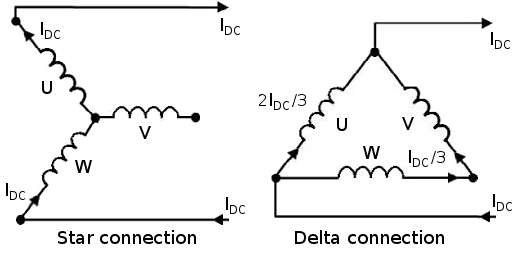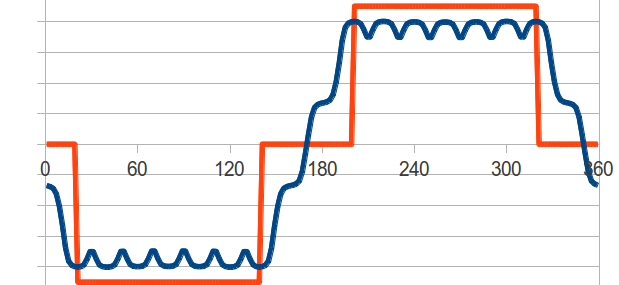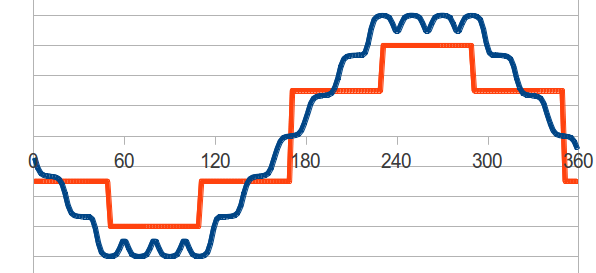# Brushless DC machine

Discussion about star- and delta-connected BLDC machines.

The brushless DC (BLDC) machine is widely used in various industrial applications due to its high energy density and low maintenance requirements. It is similar in the design compared with the BLAC machine, but differs with regard to its control. The BLDC machine is trapezoidally excited and the armature windings are fed with almost rectangular currents. The BLDC machine can be either star- or delta-connected, with both having approximately the same torque capability.

Figure 1 shows the characteristic current distribution in star- and delta-connected BLDC machines. Both are fed with rectangular line currents of 120 electrical degrees width. In a BLDC machine, the line currents are commutated every 60 electrical degrees, resulting in the current waveforms shown in Figures 2 and 3 respectively.Fig. 1 Current distribution in star- and delta-connected BLDC machines at a given time.

## Star-connected BLDC machines

In a star-connected BLDC machine, only two phases are conducting current at a given time, refer to Figure 1. The star-connected BLDC machine is designed with a magnet angle of almost 180 electrical degrees. In such a way, the phase back-EMF is almost constant during a period of 120 electrical degrees, refer to Fig. 2. The interaction between phase current and phase back-EMF results in a constant torque output with potentially low torque ripple. Torque ripple is caused by the slotting effect and the non-ideal current commutations, or when using an advance angle larger than zero.Fig. 2 Example of phase current (red) and phase back-EMF (blue) waveforms of a star-connected BLDC machine operated with an advance angle of 0°.

The conductor losses of a star-connected BLDC machine are calculated as a function of the phase resistance $R_{ph}$ and DC current $I_{DC}$ as:

$$2*R_{ph}*I_{DC}^2$$

## Delta-connected BLDC machines

In a delta-connected BLDC machine, all three phases are conducting current at a given time (when fed with the same rectangular line currents of 120 electrical degrees width as the star-connected BLDC machine). Figure 1 indicates that approximately two thirds of the current flow in one winding, while one third of the current flows in the other two series-connected windings. By choosing a magnet angle of 120 electrical degrees, there are basically no third harmonics in the airgap flux density that could cause undesired circulating currents in the delta connection. The smaller magnet angle as compared to the star-connected BLDC machine, allows to reduce the thickness of the stator back accordingly. Figure 3 shows that the phase current and phase back-EMF waveforms are more sinusoidal than those of the star-connected BLDC machine.Fig. 3 Example of phase current (red) and phase back-EMF (blue) waveforms of a delta-connected BLDC machine operated with an advance angle of 0°.

The conductor losses of a delta-connected BLDC machine can be calculated as:

$$2/3*R_{ph}*I_{DC}^2$$

## BLDC simulations

The open-circuit simulation of a BLDC machine is exactly the same as that of a BLAC machine, since there is no current flowing in the winding coils.

The load simulation can be run with either star or delta-connection of the three phase windings. In a simulation, the line currents are often assumed to be ideal square-waves of 120 electrical degrees width, characterized by the DC current and the current advance angle. The advance angle is normally in the range between 0 electrical degrees (current in phase with back-EMF) and 90 electrical degrees. In practice, the phase inductances and the non-ideal current commutations will cause a phase lag of the current which is counteracted by increasing the current advance angle. However, in a simulation that assumes ideal rectangular currents it is not necessary to advance the commutation timing.

Be careful when simulating a delta-connected BLDC machine. Circulating currents due to 3rd, 9th, 15th, etc harmonics in the airgap flux density will cause additional copper and iron losses. These circulating currents and additional losses are often not simulated, which might result in an overestimation of the machine performance if these harmonics are present.

A star-connected BLDC machine with rectangular line currents of 180 electrical degrees width has the same current distribution as a delta-connected BLDC machine with rectangular line currents of 120 electrical degrees width. Consequently, a 180° conduction star-connected BLDC machine can be simulated in Emetor as a delta-connected BLDC machine!